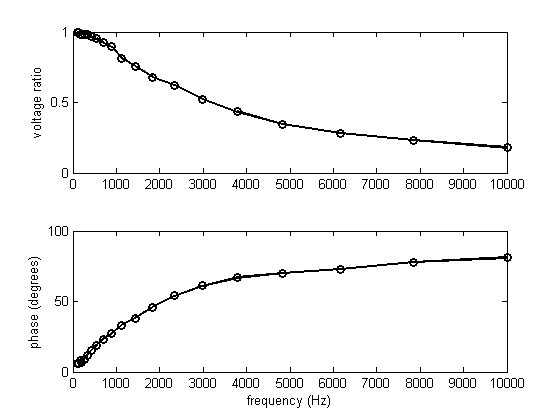# Lab Demo 4

Download MATLAB files: demo4.zip

This MATLAB script controls the function generator and oscilloscope to measure the response of an RC circuit to an input sine wave as a function of frequency.

The circuit under test is shown below.The routine get_data.m uses the oscilloscope to measure the amplitudes of the input and output signals and the phase difference between input and output.

The routine instr_open.m opens the function generator (fg) and oscilloscope (os) objects. The routine instr_close.m closes all open instrument control objects.

The autoscale function of the oscilloscope does not always work properly at low frequencies. The MATLAB code could be improved to detect and correct that situation.

## Open function generator and scope

```instr_open;

% Set fg output load
fprintf(fg,'Output:Load INF');
```
```identification: AGILENT TECHNOLOGIES,MSO7012B,MY49520152,06.00.0004
identification: HEWLETT-PACKARD,33120A,0,8.0-5.0-1.0
```

## take data

```npts = 20;
freq = logspace(2,4,npts)';
y = zeros(npts,5);
for k=1:npts
fprintf(fg,'FREQ %g',freq(k));
y(k,:) = get_data(os);
end
```

## show data

```subplot(2,1,1);
yratio = y(:,4);
yphase = y(:,5);
plot(freq,yratio,'ko-','LineWidth',2);
ylabel('voltage ratio');
subplot(2,1,2);
plot(freq,yphase,'ko-','LineWidth',2);
xlabel('frequency (Hz)');
ylabel('phase (degrees)');
```## look for 45-degree phase shift

```idx = find(yphase>30 & yphase<60);
fz = interp1(yphase(idx),freq(idx),45);
fprintf(fg,'FREQ %g',fz);
get_data(os);
```
```freq 1786 Hz vratio 0.679426 phase 45 deg
```

## compare time constant to RC

```fprintf('time constant %g usec\n',1e6/(2*pi*fz));
R = 3.8e3;
C = 0.022e-6;
tau = R*C;
fprintf('R %g ohms C %g uF tau %g usec.\n',R,C*1e6,tau*1e6);
```
```time constant 89.2292 usec
R 3800 ohms C 0.022 uF tau 83.6 usec.
```

## Close all instruments

```instr_close
clear
close all
```